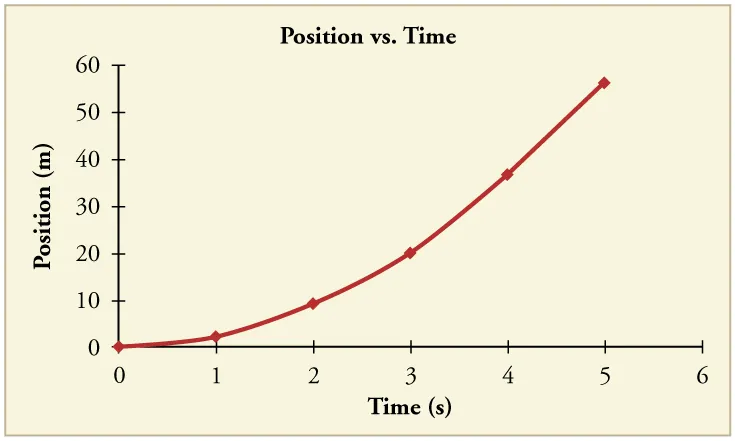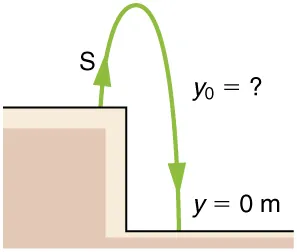College Physics 2e

# Chapter 2

### Problems & Exercises

1.

(a) 7 m

(b) 7 m

(c) $+7 m+7 m$

3.

a.

b.

c.

5.

(a) $3.0 ×104 m/s3.0 ×104 m/s$

(b) 0 m/s

7.

$2 × 10 7 years 2 × 10 7 years$

9.

$34 . 689 m/s = 124 . 88 km/h 34 . 689 m/s = 124 . 88 km/h$

11.

(a) $40.0 km/h40.0 km/h$

(b) 34.3 km/h, $25º S of E.25º S of E.$

(c) $average speed=3.20 km/h,v-=0.average speed=3.20 km/h,v-=0.$

13.

384,000 km

15.

(a) $6.61×1015rev/s6.61×1015rev/s$

(b) 0 m/s

16.

$4 . 29 m/s 2 4 . 29 m/s 2$

18.

(a) $1.43 s1.43 s$

(b) $−2.50m/s2−2.50m/s2$

20.

(a) $10.8m/s10.8m/s$

(b)21.

38.9 m/s (about 87 miles per hour)

23.

(a) $16.5 s16.5 s$

(b) $13.5 s13.5 s$

(c) $−2.68 m/s2−2.68 m/s2$

25.

(a) $20.0 m20.0 m$

(b) $−1.00 m/s−1.00 m/s$

(c) This result does not really make sense. If the runner starts at 9.00 m/s and decelerates at $2.00 m/s22.00 m/s2$, then she will have stopped after 4.50 s. If she continues to decelerate, she will be running backwards.

27.

$0 . 799 m 0 . 799 m$

29.

(a) $28.0 m/s28.0 m/s$

(b) $50.9 s50.9 s$

(c) 7.68 km to accelerate and 713 m to decelerate

31.

(a) $51.4m51.4m$

(b) $17.1 s17.1 s$

33.

(a) $−80.4m/s2−80.4m/s2$

(b) $9.33×10−2 s9.33×10−2 s$

35.

(a) $7.7 m/s7.7 m/s$

(b) $−15×102m/s2−15×102m/s2$. This is about 3 times the deceleration of the pilots, who were falling from thousands of meters high!

37.

(a) $32.6 m/s232.6 m/s2$

(b) $162 m/s162 m/s$

(c) $v>vmaxv>vmax$, because the assumption of constant acceleration is not valid for a dragster. A dragster changes gears, and would have a greater acceleration in first gear than second gear than third gear, etc. The acceleration would be greatest at the beginning, so it would not be accelerating at $32.6 m/s232.6 m/s2$ during the last few meters, but substantially less, and the final velocity would be less than 162 m/s.

39.

104 s

40.

(a) $v=12.2 m/sv=12.2 m/s$; $a=4.07 m/s2a=4.07 m/s2$

(b) $v=11.2 m/sv=11.2 m/s$

41.

(a) $y1=6.28 my1=6.28 m$; $v1=10.1 m/sv1=10.1 m/s$

(b) $y2=10.1 my2=10.1 m$; $v2=5.20 m/sv2=5.20 m/s$

(c) $y3=11.5 my3=11.5 m$; $v3=0.300 m/sv3=0.300 m/s$

(d) $y4=10.4 my4=10.4 m$; $v4=−4.60 m/sv4=−4.60 m/s$

43.

$v 0 = 4 . 95 m/s v 0 = 4 . 95 m/s$

45.

(a) $a=−9.80 m/s2a=−9.80 m/s2$; $v0=13.0 m/sv0=13.0 m/s$; $y0=0 my0=0 m$

(b) $v=0m/sv=0m/s$. Unknown is distance $yy$ to top of trajectory, where velocity is zero. Use equation $v2=v02+2ay−y0v2=v02+2ay−y0$ because it contains all known values except for $yy$, so we can solve for $yy$. Solving for $yy$ gives

$v 2 − v 0 2 = 2a y − y 0 v 2 − v 0 2 2a = y − y 0 y = y 0 + v 2 − v 0 2 2a = 0 m + 0 m/s 2 − 13.0 m/s 2 2 − 9.80 m /s 2 = 8.62 m v 2 − v 0 2 = 2a y − y 0 v 2 − v 0 2 2a = y − y 0 y = y 0 + v 2 − v 0 2 2a = 0 m + 0 m/s 2 − 13.0 m/s 2 2 − 9.80 m /s 2 = 8.62 m$

Dolphins measure about 2 meters long and can jump several times their length out of the water, so this is a reasonable result.

(c) $2.65 s2.65 s$

47.(a) 8.26 m

(b) 0.717 s

49.

1.91 s

51.

(a) 94.0 m

(b) 3.13 s

53.

(a) -70.0 m/s (downward)

(b) 6.10 s

55.

(a) $19.6 m19.6 m$

(b) $18.5 m18.5 m$

57.

(a) 305 m

(b) 262 m, -29.2 m/s

(c) 8.91 s

59.

(a) $115 m/s115 m/s$

(b) $5.0 m/s25.0 m/s2$

61.
$v = ( 11.7 − 6.95 ) × 10 3 m ( 40 . 0 – 20 .0 ) s = 238 m/s v = ( 11.7 − 6.95 ) × 10 3 m ( 40 . 0 – 20 .0 ) s = 238 m/s$
63.65.

(a) 6 m/s

(b) 12 m/s

(c) $3 m/s23 m/s2$

(d) 10 s

67.

(a) Car A is traveling faster at the checkpoint because it must go past the speed of car B to reach the same distance.

(b) i. Yes, the equation is consistent with the answer because the speed of car A is only a constant away from the correct answer. ii. Yes, the equation makes sense because $V=2 V 0 V=2 V 0$.

(c)

Order a print copy

As an Amazon Associate we earn from qualifying purchases.